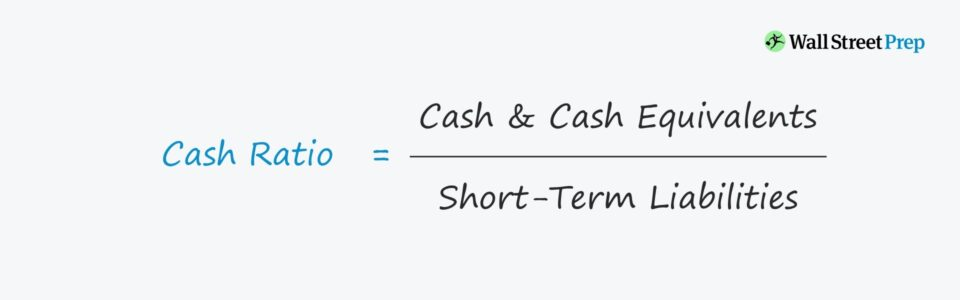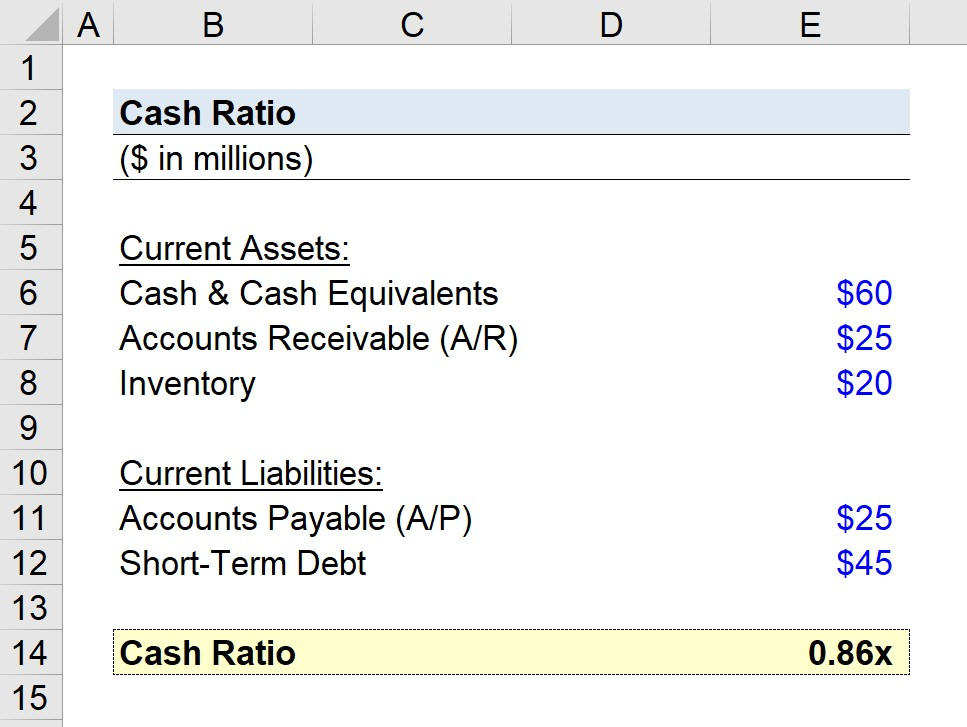Welcome to Wall Street Prep! Use code at checkout for 15% off.# Cash Ratio

Guide to Understanding the Cash Ratio## How to Calculate the Cash Ratio

The cash ratio is a measure of short-term liquidity, similar to the current ratio and quick ratio.

The formula components consist of:

By dividing a company’s most liquid cash and equivalents by the value of its short-term debt (i.e. coming due within the coming year), the ratio depicts a company’s ability to cover its near-term debt burden.

While cash is straightforward, cash equivalents include the following:

As for short-term liabilities, two common examples would be the following:

## Cash Ratio Formula

The formula for calculating the cash ratio is as follows.

###### Formula
• Cash Ratio = Cash and Cash Equivalents / Short-Term Liabilities

## How to Interpret the Cash Ratio

If the cash ratio is equal to or greater than one, the company is most likely in good health and not at risk of default — as the company has sufficient highly liquid, short-term assets to cover its short-term liabilities.

But if the ratio is less than one, that means the company’s cash and equivalents are not enough to cover upcoming spending outflows, which creates the need for easily liquidated assets (e.g. inventory, accounts receivable).

• Low Ratio → Company might have taken on too much of a debt burden, creating more risk of default.
• High Ratio → Company appears more capable of paying off short-term liabilities with its most liquid assets

## Liquidity Metrics: Cash vs. Current vs. Quick Ratio

The distinct advantage of the cash ratio is that the metric is one of the most conservative out of the commonly used liquidity measures.

• Current Ratio: For instance, the current ratio accounts for all current assets in the numerator, whereas the quick ratio only factors in cash & cash equivalents and accounts receivable.
• Quick Ratio: Since the quick ratio, or “acid test ratio”, excludes inventory, it is widely considered to be more of a strict variation of the current ratio — yet the cash ratio takes it a step further by exclusively including cash and equivalents.

Despite being relatively liquid, inventory and accounts receivable still come with some degree of uncertainty, as opposed to cash.

On the other hand, the disadvantage is that companies that hold onto cash are going to appear more financially sound than their peers that have re-invested their cash into funding future growth plans. Thus, the metric could potentially be misleading if the re-investments by a company are neglected and the ratio is taken at face value.

With that said, the metric should be used in conjunction with the current ratio and quick ratio to grasp a better picture of the liquidity position of the company.

## Cash Ratio Calculator – Excel Model Template

We’ll now move to a modeling exercise, which you can access by filling out the form below.Submitting...

## Cash Ratio Calculation Example

In our example, we’ll assume our company has the following financials:

• Cash and Equivalents = \$60 million
• Accounts Receivable (A/R) = \$25 million
• Inventory = \$20 million
• Accounts Payable = \$25 million
• Short-Term Debt = \$45 million

We can ignore the accounts receivable and inventory accounts, as mentioned earlier.

Here, our company has a short-term debt of \$45 million and \$25 million in accounts payable, which shares certain similarities with debt (i.e. vendor financing).

The cash ratio for our hypothetical company can be calculated using the formula shown below:

• Cash Ratio = \$60 million / (\$25 million + \$45 million) = 0.86x

Based on the calculated ratio, the cash and cash equivalents are inadequate to cover the liabilities with near-term maturity dates.

The 0.86x ratio implies that the company can cover ~86% of its short-term liabilities with the cash and equivalents on its balance sheet.

However, considering the accounts receivable balance of \$25 million and inventory balance of \$20 million, the company does not seem likely to default on its debt obligations or payments to its vendors in a worst-case scenario.Step-by-Step Online Course

### Everything You Need To Master Financial Modeling

Enroll in The Premium Package: Learn Financial Statement Modeling, DCF, M&A, LBO and Comps. The same training program used at top investment banks.